﻿ 基于全隐式有限差分的三元复合驱渗流模型数值计算方法

# 基于全隐式有限差分的三元复合驱渗流模型数值计算方法Numerical Computation Method for Solving Flow Model of ASP Flooding Based on Full Implicit Finite Difference

Abstract: For alkali-surfactant-polymer (ASP) flooding, there is lack of scientific mathematical model to describe the flooding mechanism, and the numerical computation has bad numerical stability and low accuracy, this paper presents a new numerical computation method based on full implicit finite difference method. Firstly, a comprehensive ASP flooding model is built with considering the influence on the physicochemical characteristic and seepage equation of oil and water and the adsorption diffusion equation which is caused by the adding of displacing agents. Then the full implicit finite difference method is applied to discretize the mechanism in time and space by block center grid system. Further, the original partial differential equations are transformed into a series of difference differential algebraic equations. At last, the problem is solved iteratively by Newton-Raphson method to get the system state and output. To verify the proposed method, a four-injection-nine-production wells example is introduced which is solved by proposed method and CMG software. Simulation result shows that the proposed method has good accuracy.

1. 引言

2. 三元复合驱数学模型

1) 地层均质，油藏等温，且吸附现象满足Langmuir等温吸附公式；

2) 驱油体系由碱、聚合物、表面活性剂组成，驱油过程为油水两相流，水相中存在碱、聚合物和表面活性剂，油相中存在表面活性剂；

3) Darcy定律适合化学剂存在情况下的油水两相；

4) 各种吸附都达到平衡，相平衡瞬间建立，且满足广义Fick定律；

$\nabla \cdot \left[\frac{K{k}_{ro}}{{B}_{o}{\mu }_{o}}\nabla \left({p}_{o}-{\rho }_{o}gh\right)\right]+{q}_{o}=\frac{\partial }{\partial t}\left[\frac{\varphi \left(1-{S}_{w}\right)}{{B}_{o}}\right],$ (1)

$\nabla \cdot \left(\frac{K{k}_{rw}}{{B}_{w}{R}_{k}{\mu }_{w}}\nabla \left({p}_{w}-{\rho }_{w}gh\right)\right)+{q}_{w}=\frac{\partial }{\partial t}\left(\frac{\varphi {S}_{w}}{{B}_{w}}\right),$ (2)

$\nabla \cdot \left(\frac{K{k}_{rw}{c}_{p}}{{B}_{w}{R}_{k}{\mu }_{w}}\nabla \left({p}_{w}-{\rho }_{w}gh\right)\right)+\nabla \cdot \left[\left({D}_{w}+{D}_{wp}\right)\frac{{\varphi }_{p}{S}_{w}}{{B}_{w}}\nabla {c}_{p}\right]+{q}_{c}=\frac{\partial }{\partial t}\left({\rho }_{r}\left(1-\varphi \right){C}_{rp}+\frac{{\varphi }_{p}{S}_{w}{c}_{p}}{{B}_{w}}\right),$ (3)

$\begin{array}{l}\nabla \cdot \left(\frac{K{k}_{rw}{c}_{ws}}{{B}_{w}{R}_{k}{\mu }_{w}}\nabla \left({p}_{w}-{\rho }_{w}gh\right)\right)+\nabla \cdot \left(\frac{K{k}_{ro}{c}_{os}}{{B}_{o}{R}_{k}{\mu }_{o}}\nabla \left({p}_{o}-{\rho }_{o}gh\right)\right)\\ \text{ }+\nabla \cdot \left[\left({D}_{o}+{D}_{os}\right)\frac{{\varphi }_{s}{S}_{o}}{{B}_{o}}\nabla {c}_{os}\right]+\nabla \cdot \left[\left({D}_{w}+{D}_{ws}\right)\frac{{\varphi }_{s}{S}_{w}}{{B}_{w}}\nabla {c}_{ws}\right]+{q}_{d}\\ =\frac{\partial }{\partial t}\left(\frac{{\varphi }_{s}{S}_{o}{c}_{os}}{{B}_{o}}+\frac{{\varphi }_{s}{S}_{w}{c}_{ws}}{{B}_{w}}+{\rho }_{r}\left(1-\varphi \right){C}_{rs}\right),\end{array}$ (4)

$\begin{array}{l}\nabla \cdot \left({D}_{OH}\frac{\varphi {S}_{w}}{{B}_{w}}\nabla {c}_{OH}\right)+\nabla \cdot \left(\frac{K{k}_{rw}{c}_{OH}}{{B}_{w}{R}_{k}{\mu }_{w}}\nabla \left({p}_{w}-{\rho }_{w}gh\right)\right)+{R}_{OH}+{q}_{e}\\ =\frac{\partial }{\partial t}\left[\frac{{K}_{a}}{{\left(1+{K}_{b}{c}_{OH}\right)}^{2}}\left(1-\varphi \right){S}_{w}{c}_{OH}+\frac{\varphi {S}_{w}{c}_{OH}}{{B}_{w}}\right],\end{array}$ (5)

 (6)

${\frac{\partial p}{\partial n}|}_{\partial \Omega }=0,\text{\hspace{0.17em}}{\text{}\frac{\partial {S}_{w}}{\partial n}|}_{\partial \Omega }=0,\text{\hspace{0.17em}}\text{}{\frac{\partial {c}_{\Theta }}{\partial n}|}_{\partial \Omega }=0,$ (7)

${q}_{o}=\left\{\begin{array}{l}-\left(1-{f}_{w}\right){q}_{out},\text{\hspace{0.17em}}\text{\hspace{0.17em}}\left(x,y,z\right)\in {\psi }_{p}\\ 0,\text{\hspace{0.17em}}\text{\hspace{0.17em}}\text{\hspace{0.17em}}\text{\hspace{0.17em}}\text{\hspace{0.17em}}\text{\hspace{0.17em}}\text{\hspace{0.17em}}\text{\hspace{0.17em}}\text{\hspace{0.17em}}\text{\hspace{0.17em}}\text{\hspace{0.17em}}\text{\hspace{0.17em}}\text{\hspace{0.17em}}\text{\hspace{0.17em}}\text{\hspace{0.17em}}\text{\hspace{0.17em}}\text{\hspace{0.17em}}\text{\hspace{0.17em}}\text{\hspace{0.17em}}\text{\hspace{0.17em}}\text{\hspace{0.17em}}\text{\hspace{0.17em}}\text{\hspace{0.17em}}\text{\hspace{0.17em}}\text{\hspace{0.17em}}\text{\hspace{0.17em}}\text{\hspace{0.17em}}\text{\hspace{0.17em}}\text{\hspace{0.17em}}\text{\hspace{0.17em}}\text{\hspace{0.17em}}\left(x,y,z\right)\notin {\psi }_{p}\end{array}$ (8)

${q}_{w}=\left\{\begin{array}{l}-{f}_{w}{q}_{out},\text{\hspace{0.17em}}\text{\hspace{0.17em}}\left(x,y,z\right)\in {\psi }_{p}\\ {q}_{in},\text{\hspace{0.17em}}\text{\hspace{0.17em}}\text{\hspace{0.17em}}\text{\hspace{0.17em}}\text{\hspace{0.17em}}\text{\hspace{0.17em}}\text{\hspace{0.17em}}\text{\hspace{0.17em}}\text{\hspace{0.17em}}\text{\hspace{0.17em}}\text{\hspace{0.17em}}\text{\hspace{0.17em}}\text{\hspace{0.17em}}\text{ }\left(x,y,z\right)\in {\psi }_{w}\\ 0,\text{\hspace{0.17em}}\text{\hspace{0.17em}}\text{\hspace{0.17em}}\text{\hspace{0.17em}}\text{\hspace{0.17em}}\text{\hspace{0.17em}}\text{\hspace{0.17em}}\text{\hspace{0.17em}}\text{\hspace{0.17em}}\text{\hspace{0.17em}}\text{\hspace{0.17em}}\text{\hspace{0.17em}}\text{\hspace{0.17em}}\text{\hspace{0.17em}}\text{\hspace{0.17em}}\text{\hspace{0.17em}}\text{ }\text{\hspace{0.17em}}\left(x,y,z\right)\notin {\psi }_{p}\cup {\psi }_{w}\end{array}$ (9)

${q}_{c}=\left\{\begin{array}{l}{q}_{w}{c}_{p},\text{\hspace{0.17em}}\text{\hspace{0.17em}}\text{\hspace{0.17em}}\text{\hspace{0.17em}}\text{\hspace{0.17em}}\text{\hspace{0.17em}}\text{\hspace{0.17em}}\text{\hspace{0.17em}}\text{ }\left(x,y,z\right)\in {\psi }_{p}\\ {q}_{w}{c}_{pin},\text{\hspace{0.17em}}\text{\hspace{0.17em}}\text{\hspace{0.17em}}\text{\hspace{0.17em}}\text{\hspace{0.17em}}\text{\hspace{0.17em}}\left(x,y,z\right)\in {\psi }_{w}\\ 0,\text{\hspace{0.17em}}\text{\hspace{0.17em}}\text{\hspace{0.17em}}\text{\hspace{0.17em}}\text{\hspace{0.17em}}\text{\hspace{0.17em}}\text{\hspace{0.17em}}\text{\hspace{0.17em}}\text{\hspace{0.17em}}\text{\hspace{0.17em}}\text{\hspace{0.17em}}\text{}\text{\hspace{0.17em}}\text{\hspace{0.17em}}\text{\hspace{0.17em}}\text{\hspace{0.17em}}\text{ }\left(x,y,z\right)\notin {\psi }_{p}\cup {\psi }_{w}\end{array}$ (10)

${q}_{d}=\left\{\begin{array}{l}{q}_{w}{c}_{ws}+{q}_{o}{c}_{os},\text{\hspace{0.17em}}\text{\hspace{0.17em}}\left(x,y,z\right)\in {\psi }_{p}\\ {q}_{w}{c}_{sin},\text{\hspace{0.17em}}\text{\hspace{0.17em}}\text{\hspace{0.17em}}\text{\hspace{0.17em}}\text{}\text{\hspace{0.17em}}\text{\hspace{0.17em}}\text{\hspace{0.17em}}\text{ }\left(x,y,z\right)\in {\psi }_{w}\\ 0,\text{\hspace{0.17em}}\text{\hspace{0.17em}}\text{\hspace{0.17em}}\text{\hspace{0.17em}}\text{\hspace{0.17em}}\text{\hspace{0.17em}}\text{\hspace{0.17em}}\text{\hspace{0.17em}}\text{\hspace{0.17em}}\text{\hspace{0.17em}}\text{}\text{\hspace{0.17em}}\text{\hspace{0.17em}}\text{\hspace{0.17em}}\text{\hspace{0.17em}}\text{\hspace{0.17em}}\text{\hspace{0.17em}}\left(x,y,z\right)\notin {\psi }_{p}\cup {\psi }_{w}\end{array}$ (11)

${q}_{e}=\left\{\begin{array}{l}{q}_{w}{c}_{OH},\text{\hspace{0.17em}}\text{\hspace{0.17em}}\text{\hspace{0.17em}}\text{\hspace{0.17em}}\text{\hspace{0.17em}}\text{\hspace{0.17em}}\text{\hspace{0.17em}}\text{ }\text{\hspace{0.17em}}\left(x,y,z\right)\in {\psi }_{p}\\ {q}_{w}{c}_{OHin},\text{\hspace{0.17em}}\text{\hspace{0.17em}}\text{\hspace{0.17em}}\text{\hspace{0.17em}}\text{\hspace{0.17em}}\text{\hspace{0.17em}}\left(x,y,z\right)\in {\psi }_{w}\\ 0,\text{\hspace{0.17em}}\text{\hspace{0.17em}}\text{\hspace{0.17em}}\text{\hspace{0.17em}}\text{\hspace{0.17em}}\text{\hspace{0.17em}}\text{\hspace{0.17em}}\text{\hspace{0.17em}}\text{\hspace{0.17em}}\text{\hspace{0.17em}}\text{}\text{\hspace{0.17em}}\text{\hspace{0.17em}}\text{ }\text{ }\text{\hspace{0.17em}}\text{ }\text{\hspace{0.17em}}\left(x,y,z\right)\notin {\psi }_{p}\cup {\psi }_{w}\end{array}$ (12)

${B}_{o}={B}_{or}/\left[1+{C}_{o}\left(p-{p}_{r}\right)\right],$ (13)

${B}_{w}={B}_{wr}/\left[1+{C}_{w}\left(p-{p}_{r}\right)\right],$ (14)

${r}_{o}=\frac{K{k}_{ro}}{{B}_{o}{\mu }_{o}},\text{\hspace{0.17em}}{r}_{w}=\frac{K{k}_{rw}}{{B}_{w}{R}_{k}{\mu }_{w}}$ (15)

${f}_{w}\left(x,y,z,t\right)$ 为含水率是状态 $p,{S}_{w},{c}_{p},{c}_{s},{c}_{OH}$ 的函数，其定义为 

${f}_{w}=\frac{{r}_{w}}{{r}_{w}+{r}_{o}},$ (16)

${c}_{s}=\frac{{q}_{w}{c}_{ws}+{q}_{o}{c}_{os}}{{q}_{w}+{q}_{o}},$ (17)

3. 全隐式有限差分求解

3.1. 全隐式有限差分离散化

${x}_{i,j,k}=x\left({x}_{i},{y}_{j},{z}_{k}\right)\text{.}$ (18)

$\frac{\partial x}{\partial t}=\frac{{x}^{n+1}-{x}^{n}}{\Delta {t}^{n}},$ (19)

$\frac{\partial x}{\partial x}=\frac{{x}_{i+1/2,j,k}-{x}_{i-1/2,j,k}}{\Delta x},$ (20)

$\frac{\partial x}{\partial y}=\frac{{x}_{i,j+1/2,k}-{x}_{i,j-1/2,k}}{\Delta y},$ (21)

$\frac{\partial x}{\partial z}=\frac{{x}_{i,j,k+1/2}-{x}_{i,j,k-1/2}}{\Delta z},$ (22)Figure 1. Schematic diagram of grid division for finite difference

${G}^{n}={F}^{n+1}\left({\stackrel{˜}{x}}^{n+1}\right)+{W}^{n+1}\left({\stackrel{˜}{x}}^{n+1},{u}^{n}\right)-\left[{A}^{n+1}\left({\stackrel{˜}{x}}^{n+1}\right)-{A}^{n}\left({\stackrel{˜}{x}}^{n}\right)\right]=0,$ (23)

$\stackrel{˜}{x}={\left[{\stackrel{˜}{x}}_{1,1,1}^{\text{T}},\cdots ,{\stackrel{˜}{x}}_{i,j,k}^{\text{T}},\cdots ,{\stackrel{˜}{x}}_{{n}_{x},{n}_{y},{n}_{z}}^{\text{T}}\right]}^{\text{T}}\in {R}^{{n}_{x}×{n}_{y}×{n}_{z}×3}$ 表示离散的状态向量， $u={\left[{u}_{i,j}|{u}_{i,j}={c}_{\Theta in,i,j},\left(i,j\right)\in {\kappa }_{w}\right]}^{\text{T}}\in {R}^{{N}_{w}}$ 表示所有注入井的驱替剂注入浓度， ${\kappa }_{w}$ 是注入井的位置集合， $F$ 表示差分方程组的流动项， $W$ 表示源汇项， $A$ 表示累积项。

${t}^{n}$ 时刻网格 $\left(i,j,k\right)$ 的差分方程组 ${G}_{i,j,k}={\left[{G}_{oi,j,k},{G}_{wi,j,k},{G}_{pi,j,k},{G}_{si,j,k},{G}_{OHi,j,k}\right]}^{\text{T}}$

${G}_{\Psi }{}_{i,j,k}^{n}={F}_{\Psi }{}_{i,j,k}^{n+1}+{W}_{\Psi }{}_{i,j,k}^{n+1}-{A}_{\Psi }{}_{i,j,k}^{n+1}+{A}_{\Psi }{}_{i,j,k}^{n}=0,\Psi =\left\{w,o,OH,s,p\right\},$ (24)

$\begin{array}{c}{F}_{Ζ}{}_{i,j,k}^{n+1}=\Delta y\Delta z\left[\frac{{r}_{Ζ}{}_{i+\frac{1}{2},j,k}^{n+1}}{\Delta x}\left({E}_{i+1,j,k}^{n+1}-{E}_{i,j,k}^{n+1}\right)+\frac{{r}_{Ζ}{}_{i-\frac{1}{2},j,k}^{n+1}}{\Delta x}\left({E}_{i-1,j,k}^{n+1}-{E}_{i,j,k}^{n+1}\right)\right]\\ \text{\hspace{0.17em}}\text{\hspace{0.17em}}+\Delta x\Delta z\left[\frac{{r}_{Ζ}{}_{i,j+\frac{1}{2},k}^{n+1}}{\Delta y}\left({E}_{i,j+1,k}^{n+1}-{E}_{i,j,k}^{n+1}\right)+\frac{{r}_{Ζ}{}_{i,j-\frac{1}{2},k}^{n+1}}{\Delta y}\left({E}_{i,j-1,k}^{n+1}-{E}_{i,j,k}^{n+1}\right)\right]\\ \text{\hspace{0.17em}}\text{\hspace{0.17em}}+\Delta x\Delta y\left[\frac{{r}_{Ζ}{}_{i,j,k+\frac{1}{2}}^{n+1}}{\Delta z}\left({E}_{i,j,k+1}^{n+1}-{E}_{i,j,k}^{n+1}\right)+\frac{{r}_{Ζ}{}_{i,j,k-\frac{1}{2}}^{n+1}}{\Delta z}\left({E}_{i,j,k-1}^{n+1}-{E}_{i,j,k}^{n+1}\right)\right],\end{array}$ (25)

$\begin{array}{c}{F}_{\Theta }{}_{i,j,k}^{n+1}=\Delta y\Delta z\left[\frac{{r}_{d\Theta }{}_{i+\frac{1}{2},j,k}^{n+1}}{\Delta x}\left({c}_{\Theta }{}_{i+1,j,k}^{n+1}-{c}_{\Theta }{}_{i,j,k}^{n+1}\right)+\frac{{r}_{d\Theta }{}_{i-\frac{1}{2},j,k}^{n+1}}{\Delta x}\left({c}_{\Theta }{}_{i-1,j,k}^{n+1}-{c}_{\Theta }{}_{i,j,k}^{n+1}\right)\right]\\ \text{\hspace{0.17em}}\text{\hspace{0.17em}}+\Delta x\Delta z\left[\frac{{r}_{d\Theta }{}_{i,j+\frac{1}{2},k}^{n+1}}{\Delta y}\left({c}_{\Theta }{}_{i,j+1,k}^{n+1}-{c}_{\Theta }{}_{i,j,k}^{n+1}\right)+\frac{{r}_{d\Theta }{}_{i,j-\frac{1}{2},k}^{n+1}}{\Delta y}\left({c}_{\Theta }{}_{i,j-1,k}^{n+1}-{c}_{\Theta }{}_{i,j,k}^{n+1}\right)\right]\\ \text{\hspace{0.17em}}\text{\hspace{0.17em}}+\Delta x\Delta y\left[\frac{{r}_{d\Theta }{}_{i,j,k+\frac{1}{2}}^{n+1}}{\Delta z}\left({c}_{\Theta }{}_{i,j,k+1}^{n+1}-{c}_{\Theta }{}_{i,j,k}^{n+1}\right)+\frac{{r}_{d\Theta }{}_{i,j,k-\frac{1}{2}}^{n+1}}{\Delta z}\left({c}_{\Theta }{}_{i,j,k-1}^{n+1}-{c}_{\Theta }{}_{i,j,k}^{n+1}\right)\right]\\ \text{ }\text{\hspace{0.17em}}\text{\hspace{0.17em}}+\Delta y\Delta z\left[\frac{{r}_{c\Theta }{}_{i+\frac{1}{2},j,k}^{n+1}}{\Delta x}\left({E}_{i+1,j,k}^{n+1}-{E}_{i,j,k}^{n+1}\right)+\frac{{r}_{c\Theta }{}_{i-\frac{1}{2},j,k}^{n+1}}{\Delta x}\left({E}_{i-1,j,k}^{n+1}-{E}_{i,j,k}^{n+1}\right)\right]\end{array}$

$\begin{array}{c}\text{ }+\Delta x\Delta z\left[\frac{{r}_{c\Theta }{}_{i,j+\frac{1}{2},k}^{n+1}}{\Delta y}\left({E}_{i,j+1,k}^{n+1}-{E}_{i,j,k}^{n+1}\right)+\frac{{r}_{c\Theta }{}_{i,j-\frac{1}{2},k}^{n+1}}{\Delta y}\left({E}_{i,j-1,k}^{n+1}-{E}_{i,j,k}^{n+1}\right)\right]\\ \text{ }+\Delta x\Delta y\left[\frac{{r}_{c\Theta }{}_{i,j,k+\frac{1}{2}}^{n+1}}{\Delta z}\left({E}_{i,j,k+1}^{n+1}-{E}_{i,j,k}^{n+1}\right)+\frac{{r}_{c\Theta }{}_{i,j,k-\frac{1}{2}}^{n+1}}{\Delta z}\left({E}_{i,j,k-1}^{n+1}-{E}_{i,j,k}^{n+1}\right)\right].\end{array}$ (26)

${W}_{o}{}_{i,j,k}^{n+1}={q}_{o}{}_{i,j,k}^{n+1}=\left\{\begin{array}{l}-\left(1-{f}_{w}{}_{i,j,k}^{n+1}\right){q}_{out}{}_{i,j}^{n},\text{\hspace{0.17em}}\text{\hspace{0.17em}}\left(x,y,z\right)\in {\psi }_{p}\\ 0,\text{\hspace{0.17em}}\text{\hspace{0.17em}}\text{\hspace{0.17em}}\text{\hspace{0.17em}}\text{\hspace{0.17em}}\text{\hspace{0.17em}}\text{\hspace{0.17em}}\text{\hspace{0.17em}}\text{\hspace{0.17em}}\text{\hspace{0.17em}}\text{\hspace{0.17em}}\text{\hspace{0.17em}}\text{\hspace{0.17em}}\text{\hspace{0.17em}}\text{\hspace{0.17em}}\text{\hspace{0.17em}}\text{\hspace{0.17em}}\text{\hspace{0.17em}}\text{\hspace{0.17em}}\text{\hspace{0.17em}}\text{\hspace{0.17em}}\text{}\text{ }\text{}\text{\hspace{0.17em}}\text{\hspace{0.17em}}\text{\hspace{0.17em}}\text{\hspace{0.17em}}\text{\hspace{0.17em}}\text{\hspace{0.17em}}\text{\hspace{0.17em}}\text{\hspace{0.17em}}\left(x,y,z\right)\notin {\psi }_{p}\end{array}$ (27)

${W}_{w}{}_{i,j,k}^{n+1}={q}_{w}{}_{i,j,k}^{n+1}=\left\{\begin{array}{l}-{f}_{w}{}_{i,j,k}^{n+1}{q}_{out}{}_{i,j}^{n},\text{\hspace{0.17em}}\text{ }\text{\hspace{0.17em}}\text{ }\left(x,y,z\right)\in {\psi }_{p}\\ {q}_{in}{}_{i,j}^{n},\text{\hspace{0.17em}}\text{\hspace{0.17em}}\text{\hspace{0.17em}}\text{\hspace{0.17em}}\text{\hspace{0.17em}}\text{\hspace{0.17em}}\text{\hspace{0.17em}}\text{\hspace{0.17em}}\text{\hspace{0.17em}}\text{\hspace{0.17em}}\text{}\text{\hspace{0.17em}}\text{\hspace{0.17em}}\text{\hspace{0.17em}}\left(x,y,z\right)\in {\psi }_{w}\\ 0,\text{\hspace{0.17em}}\text{\hspace{0.17em}}\text{\hspace{0.17em}}\text{\hspace{0.17em}}\text{\hspace{0.17em}}\text{\hspace{0.17em}}\text{\hspace{0.17em}}\text{\hspace{0.17em}}\text{\hspace{0.17em}}\text{\hspace{0.17em}}\text{\hspace{0.17em}}\text{\hspace{0.17em}}\text{}\text{\hspace{0.17em}}\text{\hspace{0.17em}}\text{\hspace{0.17em}}\text{\hspace{0.17em}}\text{\hspace{0.17em}}\left(x,y,z\right)\notin {\psi }_{p}\cup {\psi }_{w}\end{array}$ (28)

${W}_{p}{}_{i,j,k}^{n+1}={q}_{c}{}_{i,j,k}^{n+1}=\left\{\begin{array}{l}-{f}_{w}{}_{i,j,k}^{n+1}{q}_{out}{}_{i,j}^{n}{c}_{p}{}_{i,j,k}^{n+1},\text{\hspace{0.17em}}\text{\hspace{0.17em}}\left(x,y,z\right)\in {\psi }_{p}\\ {q}_{in}{}_{i,j}^{n}{c}_{pin}{}_{i,j}^{n},\text{\hspace{0.17em}}\text{\hspace{0.17em}}\text{}\text{ }\text{\hspace{0.17em}}\text{\hspace{0.17em}}\text{\hspace{0.17em}}\text{\hspace{0.17em}}\text{\hspace{0.17em}}\left(x,y,z\right)\in {\psi }_{w}\\ 0,\text{\hspace{0.17em}}\text{\hspace{0.17em}}\text{\hspace{0.17em}}\text{\hspace{0.17em}}\text{\hspace{0.17em}}\text{\hspace{0.17em}}\text{\hspace{0.17em}}\text{\hspace{0.17em}}\text{\hspace{0.17em}}\text{\hspace{0.17em}}\text{\hspace{0.17em}}\text{\hspace{0.17em}}\text{}\text{\hspace{0.17em}}\text{}\text{\hspace{0.17em}}\text{\hspace{0.17em}}\text{ }\text{ }\text{ }\left(x,y,z\right)\notin {\psi }_{p}\cup {\psi }_{w}\end{array}$ (29)

${W}_{s}{}_{i,j,k}^{n+1}={q}_{d}{}_{i,j,k}^{n+1}=\left\{\begin{array}{l}-{f}_{w}{}_{i,j,k}^{n+1}{q}_{out}{}_{i,j}^{n}{c}_{ws}{}_{i,j,k}^{n+1}-\left(1-{f}_{w}{}_{i,j,k}^{n+1}\right){q}_{out}{}_{i,j}^{n}{c}_{os}{}_{i,j,k}^{n+1},\text{\hspace{0.17em}}\text{\hspace{0.17em}}\left(x,y,z\right)\in {\psi }_{p}\\ {q}_{in}{}_{i,j}^{n}{c}_{sin}{}_{i,j}^{n},\text{\hspace{0.17em}}\text{}\text{\hspace{0.17em}}\text{\hspace{0.17em}}\text{\hspace{0.17em}}\text{\hspace{0.17em}}\text{\hspace{0.17em}}\text{\hspace{0.17em}}\text{\hspace{0.17em}}\text{\hspace{0.17em}}\text{\hspace{0.17em}}\text{ }\left(x,y,z\right)\in {\psi }_{w}\\ 0,\text{\hspace{0.17em}}\text{\hspace{0.17em}}\text{\hspace{0.17em}}\text{\hspace{0.17em}}\text{\hspace{0.17em}}\text{\hspace{0.17em}}\text{\hspace{0.17em}}\text{\hspace{0.17em}}\text{\hspace{0.17em}}\text{\hspace{0.17em}}\text{\hspace{0.17em}}\text{}\text{\hspace{0.17em}}\text{\hspace{0.17em}}\text{\hspace{0.17em}}\text{\hspace{0.17em}}\text{\hspace{0.17em}}\text{\hspace{0.17em}}\text{\hspace{0.17em}}\text{\hspace{0.17em}}\left(x,y,z\right)\notin {\psi }_{p}\cup {\psi }_{w}\end{array}$ (30)

${W}_{OH}{}_{i,j,k}^{n+1}={R}_{OH}{}_{i,j,k}^{n+1}+{q}_{e}{}_{i,j,k}^{n+1}=\left\{\begin{array}{l}{R}_{OH}{}_{i,j,k}^{n+1}-{f}_{w}{}_{i,j,k}^{n+1}{q}_{out}{}_{i,j}^{n}{c}_{OH}{}_{i,j,k}^{n+1},\text{\hspace{0.17em}}\text{\hspace{0.17em}}\left(x,y,z\right)\in {\psi }_{p}\\ {R}_{OH}{}_{i,j,k}^{n+1}+{q}_{in}{}_{i,j}^{n}{c}_{OHin}{}_{i,j}^{n},\text{\hspace{0.17em}}\text{\hspace{0.17em}}\text{\hspace{0.17em}}\text{}\text{\hspace{0.17em}}\text{\hspace{0.17em}}\text{\hspace{0.17em}}\text{\hspace{0.17em}}\left(x,y,z\right)\in {\psi }_{w}\\ 0,\text{\hspace{0.17em}}\text{\hspace{0.17em}}\text{\hspace{0.17em}}\text{\hspace{0.17em}}\text{\hspace{0.17em}}\text{\hspace{0.17em}}\text{\hspace{0.17em}}\text{\hspace{0.17em}}\text{\hspace{0.17em}}\text{\hspace{0.17em}}\text{\hspace{0.17em}}\text{}\text{\hspace{0.17em}}\text{\hspace{0.17em}}\text{\hspace{0.17em}}\text{\hspace{0.17em}}\text{\hspace{0.17em}}\text{\hspace{0.17em}}\left(x,y,z\right)\notin {\psi }_{p}\cup {\psi }_{w}\end{array}$ (31)

${f}_{w}{}_{i,j,k}^{n+1}=\frac{W{I}_{i,j,k}\left({r}_{wi,j,k}+{r}_{oi,j,k}\right)}{\underset{{k}^{\prime }=1}{\overset{{n}_{z}}{\sum }}W{I}_{i,j,{k}^{\prime }}\left({r}_{wi,j,{k}^{\prime }}+{r}_{oi,j,{k}^{\prime }}\right)},$ (32)

WI表示井指数  ，定义如下

$WI=\frac{2\text{π}K\Delta z}{\mathrm{ln}\frac{{d}_{e}}{{d}_{w}}+S},$ (33)

${d}_{e}\cong 0.28{\left[{\left(\frac{{K}_{y}}{{K}_{x}}\right)}^{\frac{1}{2}}\Delta {x}^{2}+{\left(\frac{{K}_{x}}{{K}_{y}}\right)}^{\frac{1}{2}}\Delta {y}^{2}\right]}^{\frac{1}{2}}/{\left(\frac{{K}_{y}}{{K}_{x}}\right)}^{\frac{1}{2}}+{\left(\frac{{K}_{x}}{{K}_{y}}\right)}^{\frac{1}{2}},$ (34)

${A}_{o}{}_{i,j,k}^{n+1}=\frac{{V}_{i,j,k}}{\Delta {t}^{n}}\frac{{\varphi }_{i,j,k}^{n+1}\left(1-{S}_{w}{}_{i,j,k}^{n+1}\right)}{{B}_{o}{}_{i,j,k}^{n+1}},{A}_{o}{}_{i,j,k}^{n}=\frac{{V}_{i,j,k}}{\Delta {t}^{n}}\frac{{\varphi }_{i,j,k}^{n}\left(1-{S}_{w}{}_{i,j,k}^{n}\right)}{{B}_{o}{}_{i,j,k}^{n}},$ (35)

${A}_{w}{}_{i,j,k}^{n+1}=\frac{{V}_{i,j,k}}{\Delta {t}^{n}}\frac{{\varphi }_{i,j,k}^{n+1}{S}_{w}{}_{i,j,k}^{n+1}}{{B}_{w}{}_{i,j,k}^{n+1}},{A}_{w}{}_{i,j,k}^{n}=\frac{{V}_{i,j,k}}{\Delta {t}^{n}}\frac{{\varphi }_{i,j,k}^{n}{S}_{w}{}_{i,j,k}^{n}}{{B}_{w}{}_{i,j,k}^{n}},$ (36)

$\begin{array}{l}{A}_{p}{}_{i,j,k}^{n+1}=\frac{{V}_{i,j,k}}{\Delta {t}^{n}}\left[{\rho }_{r}\left(1-{\varphi }_{i,j,k}^{n+1}\right){C}_{rp}{}_{i,j,k}^{n+1}+\frac{{\varphi }_{p}{}_{i,j,k}^{n+1}{S}_{w}{}_{i,j,k}^{n+1}{c}_{p}{}_{i,j,k}^{n+1}}{{B}_{w}{}_{i,j,k}^{n+1}}\right],\\ {A}_{p}{}_{i,j,k}^{n}=\frac{{V}_{i,j,k}}{\Delta {t}^{n}}\left[{\rho }_{r}\left(1-{\varphi }_{i,j,k}^{n}\right){C}_{rp}{}_{i,j,k}^{n}+\frac{{\varphi }_{p}{}_{i,j,k}^{n}{S}_{w}{}_{i,j,k}^{n}{c}_{p}{}_{i,j,k}^{n}}{{B}_{w}{}_{i,j,k}^{n}}\right],\end{array}$ (37)

$\begin{array}{l}{A}_{s}{}_{i,j,k}^{n+1}=\frac{{V}_{i,j,k}}{\Delta {t}^{n}}\left[\frac{{\varphi }_{s}{}_{i,j,k}^{n+1}{S}_{o}{}_{i,j,k}^{n+1}{c}_{os}{}_{i,j,k}^{n+1}}{{B}_{o}{}_{i,j,k}^{n+1}}+\frac{{\varphi }_{s}{}_{i,j,k}^{n+1}{S}_{w}{}_{i,j,k}^{n+1}{c}_{ws}{}_{i,j,k}^{n+1}}{{B}_{w}{}_{i,j,k}^{n+1}}+{\rho }_{r}\left(1-{\varphi }_{i,j,k}^{n+1}\right){C}_{rs}{}_{i,j,k}^{n+1}\right],\\ {A}_{s}{}_{i,j,k}^{n}=\frac{{V}_{i,j,k}}{\Delta {t}^{n}}\left[\frac{{\varphi }_{s}{}_{i,j,k}^{n}{S}_{o}{}_{i,j,k}^{n}{c}_{os}{}_{i,j,k}^{n}}{{B}_{o}{}_{i,j,k}^{n}}+\frac{{\varphi }_{s}{}_{i,j,k}^{n}{S}_{w}{}_{i,j,k}^{n}{c}_{ws}{}_{i,j,k}^{n}}{{B}_{w}{}_{i,j,k}^{n}}+{\rho }_{r}\left(1-{\varphi }_{i,j,k}^{n}\right){C}_{rs}{}_{i,j,k}^{n}\right],\end{array}$ (38)

$\begin{array}{l}{A}_{OH}{}_{i,j,k}^{n+1}=\frac{{V}_{i,j,k}}{\Delta {t}^{n}}\left[\frac{{K}_{a}}{{\left(1+{K}_{b}{c}_{OH}{}_{i,j,k}^{n+1}\right)}^{2}}\left(1-{\varphi }_{i,j,k}^{n+1}\right){S}_{w}{}_{i,j,k}^{n+1}{c}_{OH}{}_{i,j,k}^{n+1}+\frac{{\varphi }_{i,j,k}^{n+1}{S}_{w}{}_{i,j,k}^{n+1}{c}_{OH}{}_{i,j,k}^{n+1}}{{B}_{w}{}_{i,j,k}^{n+1}}\right],\\ {A}_{OH}{}_{i,j,k}^{n}=\frac{{V}_{i,j,k}}{\Delta {t}^{n}}\left[\frac{{K}_{a}}{{\left(1+{K}_{b}{c}_{OH}{}_{i,j,k}^{n}\right)}^{2}}\left(1-{\varphi }_{i,j,k}^{n}\right){S}_{w}{}_{i,j,k}^{n}{c}_{OH}{}_{i,j,k}^{n}+\frac{{\varphi }_{i,j,k}^{n}{S}_{w}{}_{i,j,k}^{n}{c}_{OH}{}_{i,j,k}^{n}}{{B}_{w}{}_{i,j,k}^{n}}\right],\end{array}$ (39)

$\frac{\partial x}{\partial x}=0,\frac{\partial x}{\partial y}=0,\frac{\partial x}{\partial z}=0,\forall \left(x,y,z\right)\in \partial \Omega .$ (40)

${x}_{0,j,k}={x}_{1,j,k},{x}_{{n}_{x}+1,j,k}={x}_{{n}_{x},j,k},j=1,2,\cdots ,{n}_{y},k=1,2,\cdots ,{n}_{z},$ (41)

${x}_{i,0,k}={x}_{i,1,k},{x}_{i,{n}_{y}+1,k}={x}_{i,{n}_{y},k},i=1,2,\cdots ,{n}_{x},k=1,2,\cdots ,{n}_{z},$ (42)

${x}_{i,j,0}={x}_{i,j,1},{x}_{i,j,{n}_{z}+1}={x}_{i,j,{n}_{z}},i=1,2,\cdots ,{n}_{x},j=1,2,\cdots ,{n}_{y}.$ (43)

${x}_{i,j,k}^{0}=x\left({x}_{i},{y}_{j},{z}_{k},0\right),i=1,\cdots ,{n}_{x},j=1,\cdots ,{n}_{y},k=1,\cdots ,{n}_{z}.$ (44)

3.2. 数学模型方程组求解

${\frac{\partial {G}^{n}}{\partial {\stackrel{˜}{x}}^{n+1}}|}_{{\stackrel{˜}{x}}^{n+1,l}}\delta {\stackrel{˜}{x}}^{n+1,l}=-{G}^{n}\left({\stackrel{˜}{x}}^{n+1},{\stackrel{˜}{x}}^{n},{u}^{n}\right),$ (45)

${\stackrel{˜}{x}}^{n+1,l+1}={\stackrel{˜}{x}}^{n+1,l}+\delta {\stackrel{˜}{x}}^{n+1,l},$ (46)

${\frac{\partial {G}^{n}}{\partial {\stackrel{˜}{x}}^{n+1}}|}_{{\stackrel{˜}{x}}^{n+1,l}}=\frac{\partial {F}^{n+1}}{\partial {\stackrel{˜}{x}}^{n+1,l}}+\frac{\partial {W}^{n+1}}{\partial {\stackrel{˜}{x}}^{n+1,l}}-\frac{\partial {A}^{n+1}}{\partial {\stackrel{˜}{x}}^{n+1,l}}.$ (47)

4. 仿真实例

4.1. 油藏描述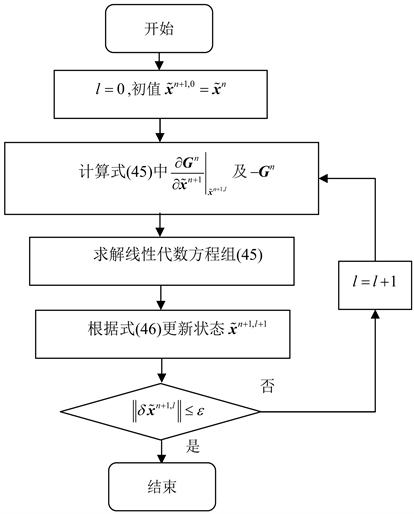Figure 2. Solution process of Newton-Raphson method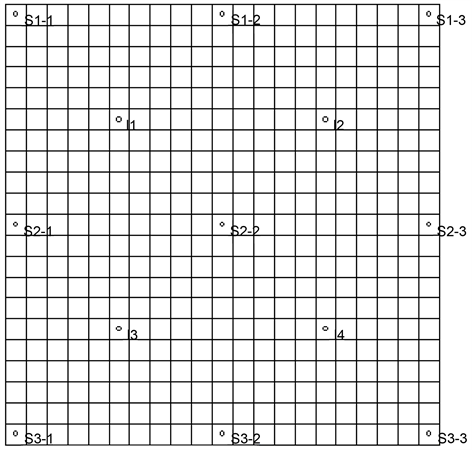Figure 3. Distribution of well position

4.2. 仿真结果

${c}_{OHin}=\left(3.7,2.8,1.3\right)\text{g}/\text{L},\text{\hspace{0.17em}}{c}_{sin}=\left(2.9,1.3,0.6\right)\text{g}/\text{L},\text{\hspace{0.17em}}{c}_{pin}=\left(2.7,1.4,1.1\right)\text{g}/\text{L}.$

5. 结论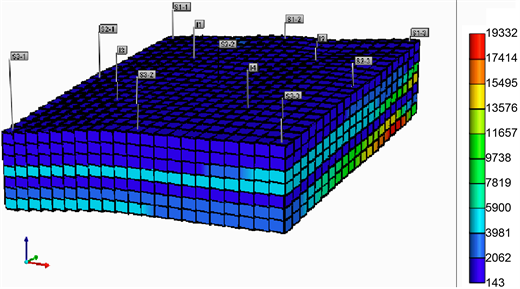Figure 4. Distribution of initial permeability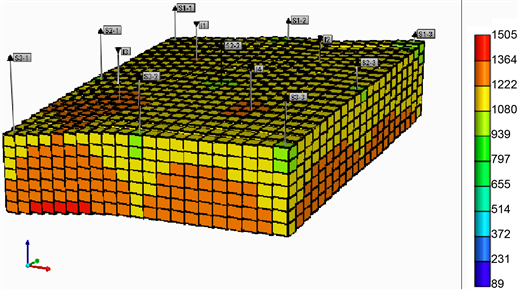Figure 5. Distribution of initial pressure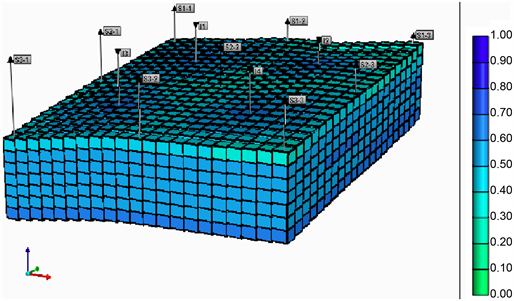Figure 6. Distribution of initial water saturation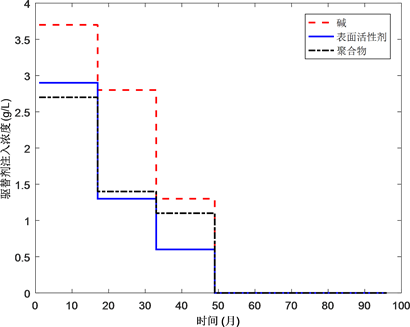(a)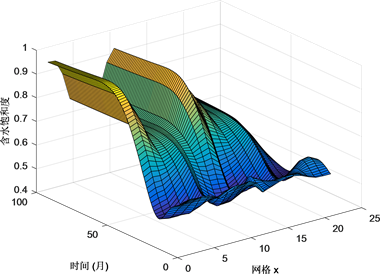(b)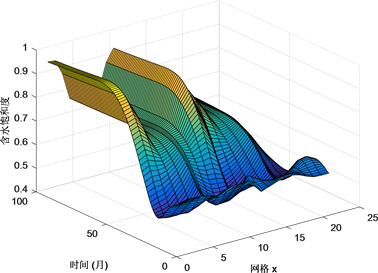(c)

Figure 7. Comparison of solution results. (a) Injection concentration; (b) Simulation result of CMG software; (c) Solution reult with proposed method in this paper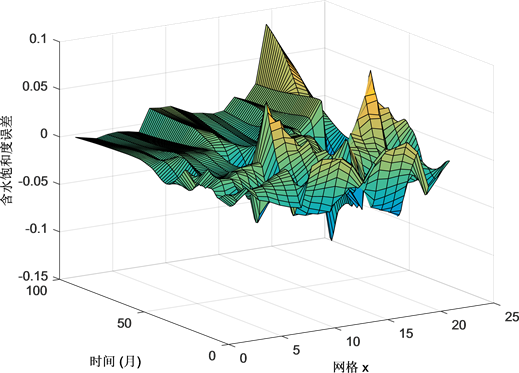Figure 8. Modeling error of water saturation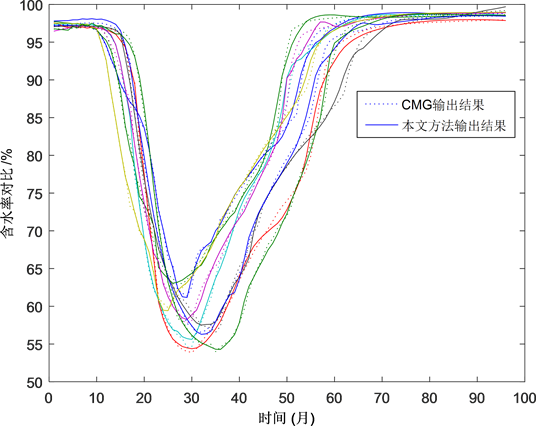Figure 9. Comparison of moisture content for nine production wellsTable 1. Liquid withdrawal amount of production wellsTable 2. Partial reservoir parameters for ASP flooding

1) 碱耗

${R}_{OH}=-\varphi {S}_{w}\frac{\partial }{\partial t}\left({r}_{1}+{r}_{2}+{r}_{3}\right),$ (48)

a) 溶液中的Na+与岩石表面的 ${\text{H}}^{\text{+}}$ 得离子交换引起的快速碱耗 ${r}_{1}$ ：该碱耗可近似表示为类似Langmuir型的吸附等温式：

${r}_{1}={r}_{1}{}^{0}\frac{{a}_{1}{c}_{OH}}{1+{a}_{1}{c}_{OH}},$ (49)

b) 原油中酸性物质引起的碱耗 ${r}_{2}$

${r}_{2}={r}_{2}\left({\text{HA}}_{w},{c}_{OH}\right),$ (50)

c) Ca2+，Mg2+离子引起的碱耗 ${r}_{3}$

${r}_{3}={r}_{3}\left({K}_{{\text{Ca}}^{\text{2+}}},{K}_{{\text{Mg}}^{\text{2+}}},{c}_{OH}\right),$ (51)

2) 表面张力

$\sigma =\sigma \left({c}_{OH},{c}_{p},{c}_{s}\right).$ (52)

3) 毛细管压力

${p}_{cow}\left({S}_{w}\right)={C}_{PC}\cdot \sqrt{\frac{\varphi }{{K}_{a}}}\cdot {\left(1-{S}_{n}\right)}^{{N}_{PC}},$ (53)

4) 表面活性剂吸附

${C}_{rs}={C}_{rs}^{0}\frac{{a}_{s}{c}_{s}}{1+{a}_{s}{c}_{s}}\left(1-{b}_{s}\frac{\text{pH}-\text{7}}{{\text{pH}}_{\text{max}}-\text{7}}\right),$ (54)

5) 聚合物的吸附量

${C}_{rp}={C}_{rp}^{\text{max}}\frac{{a}_{1}{c}_{p}}{1+{b}_{1}{c}_{p}},$ (55)

6) 相对渗透率

${K}_{rw,ro}=A\cdot {\left(1-{S}_{w}\right)}^{B}\cdot {\left({S}_{w}-C\right)}^{D},$ (56)

${R}_{k}=1+\frac{\left({R}_{k}^{\mathrm{max}}-1\right)\cdot {q}_{p}}{{q}_{p}^{\mathrm{max}}},$ (57)

7) 液相的黏度

${\mu }_{p}^{0}={\mu }_{w}\left(1+{a}_{1}{c}_{p}^{1}+{a}_{2}{c}_{p}^{2}+{a}_{3}{c}_{p}^{3}+\cdots \right),$ (58)

ASP溶液的黏度与剪切速率( $\stackrel{˙}{\gamma }$ )的关系可由Meter方程表达为

${\mu }_{\text{ASP}}={\mu }_{w}+\frac{{\mu }_{p}^{0}-{\mu }_{w}}{1+{\left[\frac{\stackrel{˙}{\gamma }}{{\stackrel{˙}{\gamma }}_{1/2}}\right]}^{{P}_{a}-1}},$ (59)

8) 残余饱和度

${N}_{c}=\frac{|\sum {}_{j}{\mu }_{j}{\stackrel{¯}{V}}_{j}|}{\sigma },$ (60)

${S}_{rj}={S}_{rj}\left({N}_{c}\right),$ (61)

 杨承志, 等. 化学驱提高石油采收率[M]. 北京: 石油工业出版社, 2007.

 程光明, 陈超, 卢珊珊, 等. 化学驱替剂的研究进展[J]. 当代化工, 2016, 45(2): 383-386.

 李树荣, 张晓东. 聚合物驱提高原油采收率的最优控制方法[M]. 东营: 中国石油大学出版社, 2013.

 李宜强, 张素梅, 刘书国, 等. 泡沫复合驱物理模拟相似原理[J]. 大庆石油学院学报, 2003, 27(2): 93-95.

 Thomas, C.P., Fleming, P.D. and Winter, W.K. (1984) A Ternary, Two-Phase, Mathematical Model of Oil Recovery With Surfactant Systems. Society of Petroleum Engineers Journal, 24, 606-616.
https://doi.org/10.2118/12934-PA

 袁士义, 杨普华. 碱复合驱数学模型[J]. 石油学报, 1994, 15(2): 76-88.

 侯健. 用流线方法模拟碱/表面活性剂/聚合物三元复合驱[J]. 石油大学学报(自然科学版), 2004, 28(1): 58-62.

 孙玉晓. 对流扩散方程的有限差分法[D]: [硕士学位论文]. 成都: 西南石油大学, 2011.

 Islam, M.N. and Azaiez, J. (2005) Fully Implicit Finite Difference Pseudo-Spectral Method for Simulating High Mobility-Ratio Miscible Displacements. International Journal for Numerical Methods in Fluids, 47, 161-183.
https://doi.org/10.1002/fld.803

 Kim, M., Song, S. and Jun, M.S. (2016) A Study of Block Chain-Based Peer-to-Peer Energy Loan Service in Smart Grid Environments. Advanced Science Letters, 22, 2543-2546.
https://doi.org/10.1166/asl.2016.7811

 刘坤, 孙建孟, 陈心宇. 水平井致密油储层近井和远井可压性研究[J]. 测井技术, 2017(4): 443-447.

 陈会娟, 李明忠, 狄勤丰, 等. 多点注汽水平井井筒出流规律数值模拟[J]. 石油学报, 2017, 38(6): 696-704.

 Quan, N., Son, N.H. and Tuan, N.Q. (2017) Minimum Volume of the Longitudinal Fin with Rectangular and Triangular Profiles by a Modified Newton-Raphson Method. International Journal of Computational Methods, 8, 1-9.
https://doi.org/10.1142/S0219876218500342

 Bahrami, P., Kazemi, P., Mahdavi, S., et al. (2016) A Novel Approach for Modeling and Optimization of Surfactant/Polymer Flooding Based on Genetic Programming Evolutionary Algorithm. Fuel, 179, 289-298.
https://doi.org/10.1016/j.fuel.2016.03.095

 Xu, L., Zhao, H., Li, Y., et al. (2018) Production Optimization of Polymer Flooding Using Improved Monte Carlo Gradient Approximation Algorithm with Constraints. Journal of Circuits Systems & Computers, 2, 167-185.

 Lei, Y., Li, S.R., Zhang, X.D., et al. (2012) Optimal Control of Polymer Flooding Based on Maximum Principle. Journal of Applied Mathematics, 1, 203-222.
https://doi.org/10.1155/2012/987975

 Ge, Y.L., Li, S.R. and Qu, K.X. (2014) A Novel Empirical Equation for Relative Permeability in Low Permeability Reservoirs. Chinese Journal of Chemical Engineering, 22, 1274-1278.
https://doi.org/10.1016/j.cjche.2014.09.031

Top